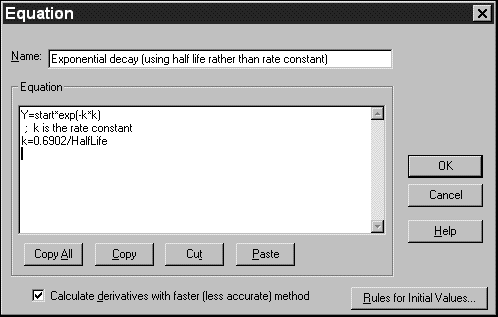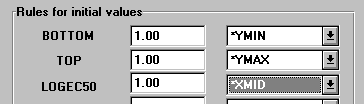KNOWLEDGEBASE - ARTICLE #1763

# Prism 3 -- What kind of equations can you enter into Prism?

Prism 3 -- User-defined equations

What kind of equations can you enter into Prism?

You don't have to use one of the classic built-in equations. You can enter your own equations into Prism, subject to these limitations:

 Limitation Explanation No implicit equations. Y must be defined as a function of X and one or more parameters. The variable Y can only appear once, on the left side of the last line of the equation. If Y also appears on the right side of the equation, you have an implicit equation, which Prism cannot handle. In many cases, you'll be able to algebraically rearrange the equation. No differential equations. With Prism, you must define Y as a function of X and one or more variables. It is not sufficient to define the derivatives. No equations with more than one X variable. Prism does not calculate multiple regression, so cannot fit models with two or more independent (X) variables. For example, Prism cannot fit a model that defines response as a function of both dose and time. No equations with more than 14 variables. Prism fits at most 14 variables, although you may use other intermediate variables when writing the equation. No discontinuous equations. If you enter a discontinuous equation (where an infinitesimal change in X can create a huge change in Y) the results of nonlinear regression may not be reliable. This is a general limitation of nonlinear regression, not specific to Prism.

Entering a user-defined equation

At the top of the parameters dialog for nonlinear regression (or simulate curve) select "More equations". Then select "Enter your own equation" to bring up the Equation dialog.You must enter a name for the equation, which will  then appear on the list of "more equations" in the nonlinear regression dialog.In entering the equation itself, follow these guidelines (similar to those of the Basic computer language).

• Variable names must not be longer than 13 characters. If you want to use two words to describe a variable, separate with the underscore character, for example Half_Life. Don't use a space, hyphen or period.
• Prism does not distinguish between upper and lower case letters in variable names.
• Use an asterisk (*) to indicate multiplication and a caret (^) to indicate power. For example, "A*B" is the product of A times B and "A^B" is A to the B power.
• Use parentheses as necessary to show the order of operations. To increase readability, substitute brackets [like this] or braces {like this}. Prism interprets parentheses, brackets, and braces identically.
• Use a single equals sign to assign a value to a variable.
• You don't need any special punctuation at the end of a statement.
• To enter a long line, type a backslash (\) at the end of the first line, then press Return and continue. Prism treats the two lines as one.
• To enter a comment, type a semicolon (;) and type text. Comments can begin anywhere on a line.
• Variable names must not be longer than 13 characters. If you want to use two words to describe a variable, separate with the underscore character, for example Half_Life. Don't use a space, hyphen or period.

You don't have to write your equation on one line. Use intermediate variables to simplify longer equations. Prism automatically distinguishes between intermediate variables and equation parameters that you can fit. If a variable is used first on the left side of an equals sign, then it is an intermediate variable. If a variable is used first on the right side of an equals sign, then it is an equation parameter.

Here are three examples of one-line equations:

Y=Bmax*X/(Kd + X)

Y=A*(X+1)

Y=Start*exp[(-0.693/Half_Life)*K]

Below is an example of a longer equation. Because K is on the left of the equals sign, Prism recognizes that it is an intermediate variable rather than a variable to be fit by nonlinear regression. Note two comments, one on a line by itself and the other on the same line with equation code.

; One-phase exponential decay
K=0.693/HalfLife ;rate constant
Y=Start*exp(-K*X)

Available functions

When you enter your equations, you can use any of the functions listed below. Don't use any of those names for your variables. (The variables j and k in the descriptions below can be any variable or expression.)

 Function Explanation abs(k) Absolute value. If k is negative, multiply by -1. arccos(k) Arccosine. Result is in radians. arcsin(k) Arcsine. Result is in radians. arctan(k) Arctangent. Result is in radians. cos(k) Cosine. K is in radians. deg(k) Converts k radians to degrees. exp(k) e to the kth power. if(condition, j, k) If the condition is true, then the result is J. Otherwise the result is k. The condition is in the form A0, sgn(k)=1. If k<0, sgn(k)= -1. If k=0, sgn(k)=0. sin(k) Sine. K is in radians. sqrt(k) Square root. tan(k) Tangent. K is in radians.

Using the IF function

Prism allows you to introduce some branching logic through use of the If  function. The syntax is:

IF (conditional expression, value if true, value if false)

You can precede a conditional expression with NOT, and can connect two conditional expressions with AND or OR. Examples of conditional expressions:

MAX>100

Ymax=Constraint

(A<B or A<C)

NOT(A<B AND A<C)

FRACTION<>1.0     )

X<=A and X>=B

Note: "<>" means not equal to. "<=" means less than or equal to. ">=" means greater than or equal to.

Here is an example.

Y= If (X<X0, Plateau, Plateau*exp(-K*X))

If X is less than X0, then Y is set equal to the variable Plateau. Otherwise Y is computed as Plateau*exp(-K*X).You may also insert a conditional expression anywhere in an equation, apart from an If function. A conditional expression evaluates as 1.0 if true and 0.0 if false. Example:

Y=(X<4)*1 + (X>=4)*10

When X is less than 4, this evaluates to 1*1 + 0*10=1. When X is greater than 4, this evaluates to 0*1+1*10=10.

Defining rules for initial values in nonlinear regression

Before it can perform nonlinear regression, Prism must have initial values for each variable in the equation. You can enter initial values at the time you fit curves, but it is helpful to define rules for generating the initial values at the time you enter a new equation. Then Prism will calculate the initial values automatically. If you don't enter rules for initial values, you will need to enter the initial values for every variable, for every data set, every time you fit data.

To define rules for initial values for user-defined equations:

While entering or editing a user-defined equation, click on the button labeled "Rules for initial values". On the Default Values dialog, enter the rule for finding the initial value of each variable. For each variable in the equation, enter a number in the first column and select a multiplier from the drop-down list in the second column.All but two choices on the drop-down list are used to multiply or divide the number you entered by a value determined from the range of the data: YMIN, YMAX, YMID, XMIN, XMAX, XMID, XMID/YMID, XMAX-XMIN, or YMAX-YMIN. The abbreviation YMIN is the minimum value of Y; YMAX is the maximum value, and YMID is the average of YMIN and YMAX. For example, if you enter "0.5" in the first column and select "YMAX" in the second column, Prism sets the initial value to half of YMAX (which differs for each data set).

The first choice on the drop-down list is "(Initial value, to be fit)". This means that the value you entered will be the initial value for all data sets. The initial value will not depend on the range of the data.

The last choice is "(Hold constant)". This sets the parameter to a constant value, that won't be fit by nonlinear regression.

You can choose "(Hold constant)" even for parameters such as specific activity or protein concentration that have different values in each experiment. In the Rules for initial value dialog, choose "(Hold constant)" but leave the value blank. Each time the equation is used, Prism will prompt for a constant value before using the equation in nonlinear regression.

Creating equation library files

When you choose an equation for nonlinear regression (or simulations), Prism lets you import from the equation library. You can add to the library by following these steps:

1. Create a new Prism project. Use the Simulate Curve analysis and enter a new equation. Pick reasonable values for the minimum and maximum X values of the curve.

2. Customize the graph so it will be clear when seen as a preview (test this by looking at the graph gallery).

3. Repeat with any number of related equations that you want to store in one file.

4. Save the file into the Equations folder within the program folder.

5. If you are creating an equation file that will be used by others, consider creating a help file that explains the equations. Using any help compiler, create a help file with the same name as the file containing the equations but with the extension hlp. Place the help file in the same folder with the Equation file.

When you choose to select an equation from a library file, Prism displays a list of all files (and templates) in the Equations folder. When you pick one of those files, it shows a list of all user-defined equations used in the file, along with a thumbnail preview of the first graph linked to each equation.  If a help file exists for the selected file, click "Help with this equation file" to open it.

## Explore the Knowledgebase

Analyze, graph and present your scientific work easily with GraphPad Prism. No coding required.